Latest Banking jobs   »   RBI Assistant Vacancy 2022   »

# Quantitative Aptitude Quiz For RBI Grade B/ ECGC PO/ SIDBI Grade A Prelims 2022- 8th April

Directions (1-5): Please read the given data carefully and answer the following questions.
The given pie chart shows the percentage distribution of students in five different school, A, B, C, D & E. Moreover, the bar graph shows the percentage of girls out of total students in each school.
Note: 1.Total students in all five school are 2500.
2. Total students in any school=Number of boys + Number of girls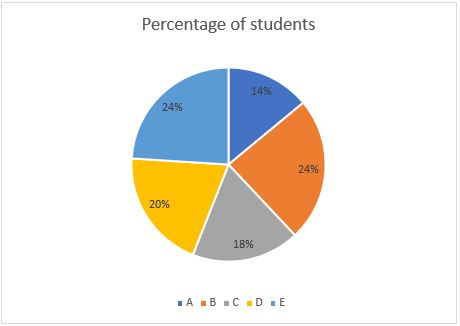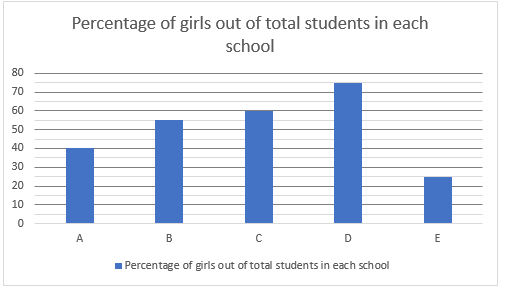Q1. Find the difference between the average number of boys to that of girls in all five schools.
(a)6
(b)9
(c)12
(d)3
(e)15

Q2. Find the respective ratio of number of boys in school A & B together to number of girls in school D & E together?
(a)35:36
(b)36:31
(c)31:36
(d)32:35
(e)35:32

Q3. Find the number of boys in school E is approximately what percent more than the total number of girls in school in A & C together?
(a)25%
(b)5%
(c)20%
(d)15%
(e)10%

Q4. If the school fee of each student of school C and D is Rs.6000 and Rs.4000 respectively, then find difference between the total fee collected from school C and D.
(a)47 lakhs
(b)51 lakhs
(c)42 lakhs
(d)55 lakhs
(e)38 lakhs

Q5. If 40%, 50% and 30% students from school A, B and E respectively use school buses to reach their school, then find the total number of students who didn’t use school bus from school A, B and E?
(a)820
(b)1030
(c)930
(d)880
(e)980

Directions (6-10): Please read the given data carefully and answer the following questions.
Sarthak invested Rs. P at 50% per annum simple interest in X bank. At the end of one – year, he withdrew all amount from the X bank and invested in Y bank at the rate of R% per annum at compound interest for two years and received Rs.14400 as total interest from the Y bank. The first year’s interest from Y bank was Rs.6000.

Q6. Find the sum of interest earned from both bank X and bank Y. (in Rs.)
(a)19400
(b)20100
(c)18600
(d)21200
(e)17900

Q7. If Sarthak invested Rs. P for three years at (R/2)% rate p.a. at compound interest , then calculate the interest received as per this scheme? (in Rs.)
(a)8510
(b)8240
(c)7760
(d)8110
(e)7280

Q8. Find the value of (62.5% of R)³-120% of P?
(a)2315
(b)2565
(c)3245
(d)3625
(e)2785

Q9. Find the difference in interest received when Rs. P invested for 2 years at R% rate per annum simple interest and at compound interest? (in Rs.)
(a)2000
(b)1600
(c)1200
(d)2400
(e)2800

Q10. Find the respective ratio of rate at which Sarthak invested his money at simple interest to that at compound interest?
(a)1:2
(b)3:2
(c)5:4
(d)4:1
(e)2:3

Directions (11-15): Please read the given data carefully and answer the following questions.
The following pie chart shows the percentage distribution of number of books published by five various division, A, B, C , D & E, of XYZ Publications. In addition to chart, table below shows the ratio of number of sold books to unsold books.
Note: 1. Total books published by division C are 1200.
2. Total books published=Sold books+Unsold books.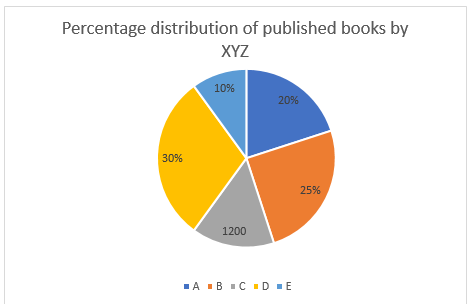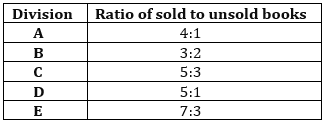Q11. Find the number of unsold books by D are what percent more/less the than the total sold books by A?
(a)82.50%
(b)78.50%
(c)74.75%
(d)62.50%
(e)68.75%

Q12. Find the respective ratio of number of sold books of C & D together to the number of unsold books of A & E together?
(a)275:56
(b)225:182
(c)47:182
(d)182: 47
(e)76:225

Q13. Find the difference between the number of sold books by D & E together and unsold books by D and E together?
(a)1975
(b)1940
(c)1890
(d)1920
(e)2010

Q14. If the ratio of defected books to perfect books is 3:2 of the unsold books under division D’s publication, then find the number of defected books?
(a)200
(b)240
(c)280
(d)320
(e)360

Q15. Find the difference between the total number of books published by A & B together and the total number of sold books by all divisions together?
(a)2270
(b)2250
(c)2190
(d)2020
(e)1990

Solutions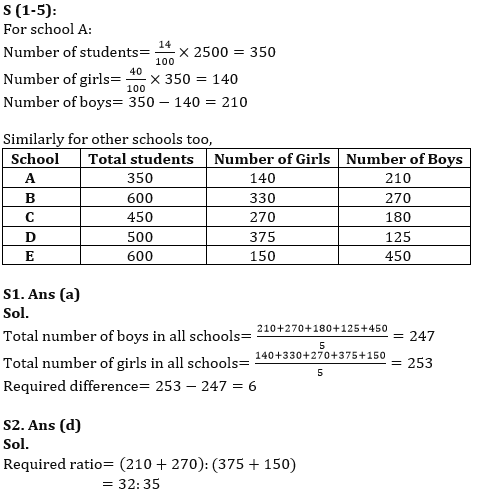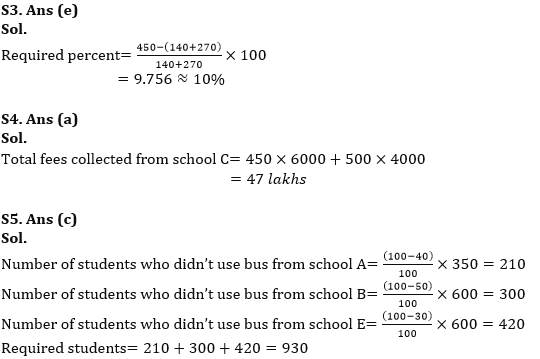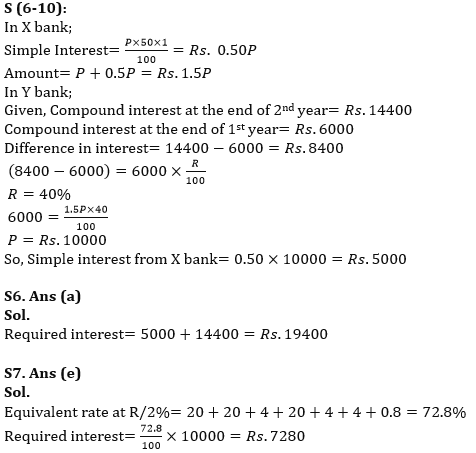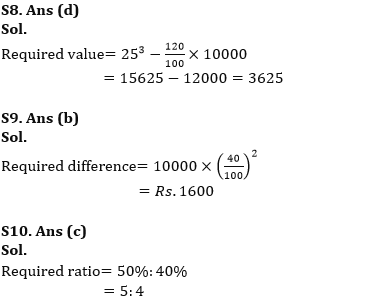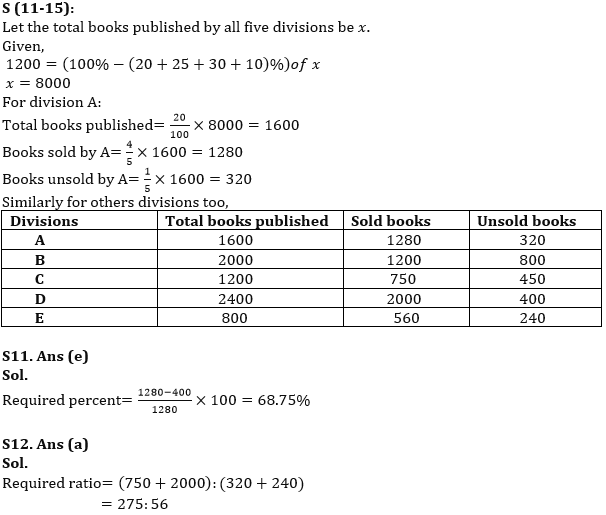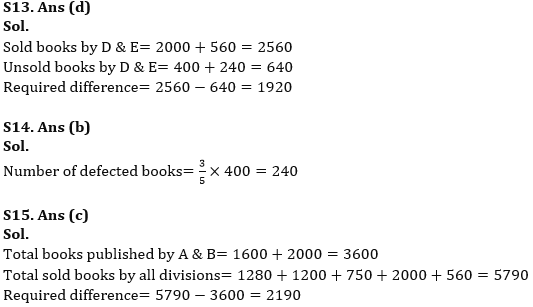#### Congratulations!Download Hindu Review of October 2021: Free PDF